Courses

# RD Sharma Solutions (Part - 2) - Ex-21.2, Mensuration - II Area of Circle, Class 7, Math Class 7 Notes | EduRev

## Class 7: RD Sharma Solutions (Part - 2) - Ex-21.2, Mensuration - II Area of Circle, Class 7, Math Class 7 Notes | EduRev

The document RD Sharma Solutions (Part - 2) - Ex-21.2, Mensuration - II Area of Circle, Class 7, Math Class 7 Notes | EduRev is a part of the Class 7 Course RD Sharma Solutions for Class 7 Mathematics.
All you need of Class 7 at this link: Class 7

#### Question 11:

The perimeter of a circle is 4πr cm. What is the area of the circle?

We have :
Given perimeter of the circle = 4πr cm = 2π (2r) cm

We know that, the perimeter of a circle = 2ππr

∴ Radius of the circle = 2r cm

Area of the circle= πr2   = π (2r)2 = 4πr2

#### Question 12:

A wire of 5024 m length is in the form of a square. It is cut and made a circle. Find the ratio of the area of the square to that of the circle.

We have:
Perimeter of the square = 5024 m = Circumference of the circle
⇒ 4 x Side of the square = 5024

∴ Side of the square = 5024/4 = 1256 m
Let the area of the square be A1 and the area of the circle be A2.
Area of the square (A1)= side x side =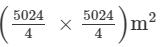Circumference of the circle = 5024 m
⇒ 2 πr = 5024 m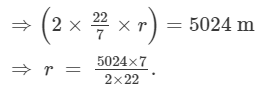Area of the circle (A2)= πr2   =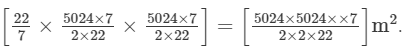∴A: A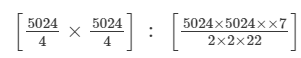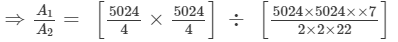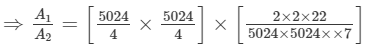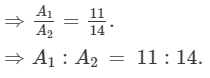Hence, the ratio of the area of the square to the area of the circle is 11:14.

#### Question 13:

The radius of a circle is 14 cm. Find the radius of the circle whose area is double of the area of the circle.

Let the area of the circle whose radius is 14 cm be A1.
Let the radius and area of the circle, whose area is twice the area of the circle A1 , be r2 and A2, respectively.
Thus,
A1 = π(r)2 = π(14)2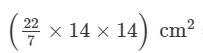= =(44×14) cm2= 616 cm2

A1 = 2 × A1 = 2 × 616 = 1232 cm2
A2 = π(r2)2 = 1232 cm2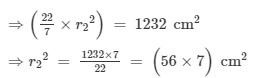⇒r2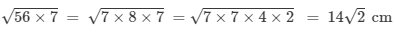Hence, the radius of the circle A2 is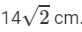#### Question 14:

The radius of one circluar field is 20 m and that of another is 48 m. Find the radius of the third circular field whose area is equal to the sum of the areas of two fields.

Let the area of the circle whose radius is 20 m be A1 , and the area of the circle whose radius
is 48 m be A2. Let A3 be the area of a circle that is equal to the sum of the areas of the two fields, with the radius of its field being r cm.
∴ A3 =  A1 + A2

A1 =  π(20)2 =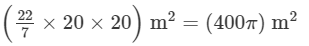A2 = π (48)2   =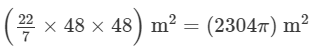A3 = A1+A2  = (400π) +(2304π) = π(400 +2304) m2
⇒A3 = π(r)2 =  π(400 +2304) m2
⇒(r)2 = (400 +2304) m2

⇒ r  =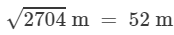#### Question 15:

The radius of one circular field is 5 m and that of the other is 13 m. Find the radius of the circular field whose area is the difference of the areas of first and second field.

Let the area of the circular field whose radius is 5 m be A1 , and the area of the circular field whose radius is 13 m be A2. Let A3 and r cm be the area and radius of the circular field, that is equal to the difference of the areas of the two fields.
∴ A3 A2 -A1

A1 =  π(5)2 = (25π) m
A2 = π (13)2 = (169 π) m2
A3 = A2−A1  = (169π) −(25 π) = 144π m2
⇒A3 = π(r)2 =  144 π m2
⇒(r)2 = 144 m2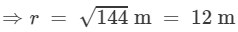Hence, the radius of the circular field is 12 m.

#### Question 16:

Two circles are drawn inside a big circle with diameters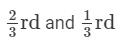of the diameter of the big circle as shown in Fig. 18. Find the area of the shaded portion, if the length of the diameter of the circle is 18 cm.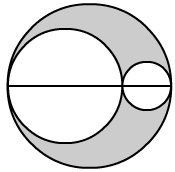Let the left circle be denoted as the 1st circle and the right circle be denoted as the 2nd circle.
Diameter of the big circle = 18 cm
Radius of the big circle = 9 cm
Diameter of the 1st circle =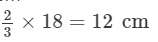Radius of the 1st circle = 6 cm
Diameter of the 2nd circle =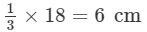Radius of the 2nd circle = 3 cm
Area of the 1st circle = π(6)2=36π cm2
Area of the 2nd circle = π(3)2=π×3×3=9π cm2
Area of the big circle = π(9)2=π×9×9=81π cm2
Area of the shaded portion = Area of the big circle - (Area of the Ist circle + Area of the IInd circle)
Area of the shaded portion = 81π−(36π+9π)=36π cm2.

#### Question 17:

In Fig. 19, the radius of quarter circular plot taken is 2 m and radius of the flower bed is 2 m. Find the area of the remaining field.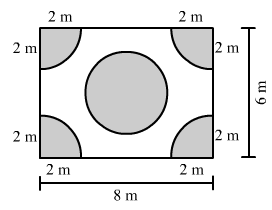#### nswer 17:

Radius of the quarter circular plot = 2 m
Area of the quarter circular plot =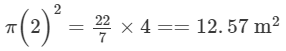Radius of each flower bed = 2 m
Area of four flower beds =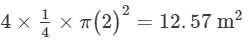Area of the rectangular region = Length × Breadth
Area of the rectangular region = 8 × 6 = 48 m2
Area of the remaining field = Area of the rectangular region - (Area of the quarter circle + Area of the four flower beds)

Area of the remaining field = [48 - (12.57 + 12.57)] m2 = 22.86 m2

#### Question 18:

Four equal circles, each of radius 5 cm, touch each other as shown in Fig. 20. Find the area included between them. (Take π = 3.14).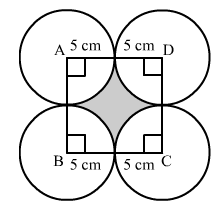Side of the square = 10 cm
Area of the square = Side × Side
Area of the square = 10×10=100 cm2

Area of the four quarter circles =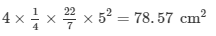Area included in them = Area of the square - Area of the four quarter circles
Area included in them = ( 100 - 78.57 ) cm2 = 21.43 cm2 .

#### Question 19:

The area of circle is 100 times the area of another circle. What is the ratio of their circumferences?

Let the area of the first circle be A1, the circumference be  C1 and the radius be r1.
Let the area of the second circle be A2 , the circumference be C2  and the radius be r2.
Thus,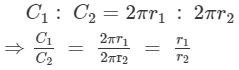We know that :

A1 =  100A2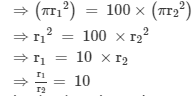Substituting the values, we get: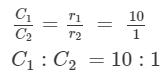Hence, the ratio of their circumferences is 10:1.

The document RD Sharma Solutions (Part - 2) - Ex-21.2, Mensuration - II Area of Circle, Class 7, Math Class 7 Notes | EduRev is a part of the Class 7 Course RD Sharma Solutions for Class 7 Mathematics.
All you need of Class 7 at this link: Class 7Use Code STAYHOME200 and get INR 200 additional OFF Use Coupon Code
All Tests, Videos & Notes of Class 7: Class 7

### Top Courses for Class 7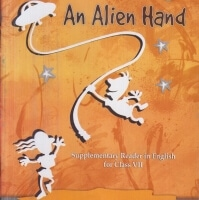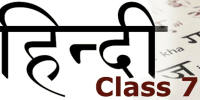## RD Sharma Solutions for Class 7 Mathematics

97 docs

### Top Courses for Class 7Track your progress, build streaks, highlight & save important lessons and more!

,

,

,

,

,

,

,

,

,

,

,

,

,

,

,

,

,

,

,

,

,

,

,

,

,

,

,

,

,

,

;